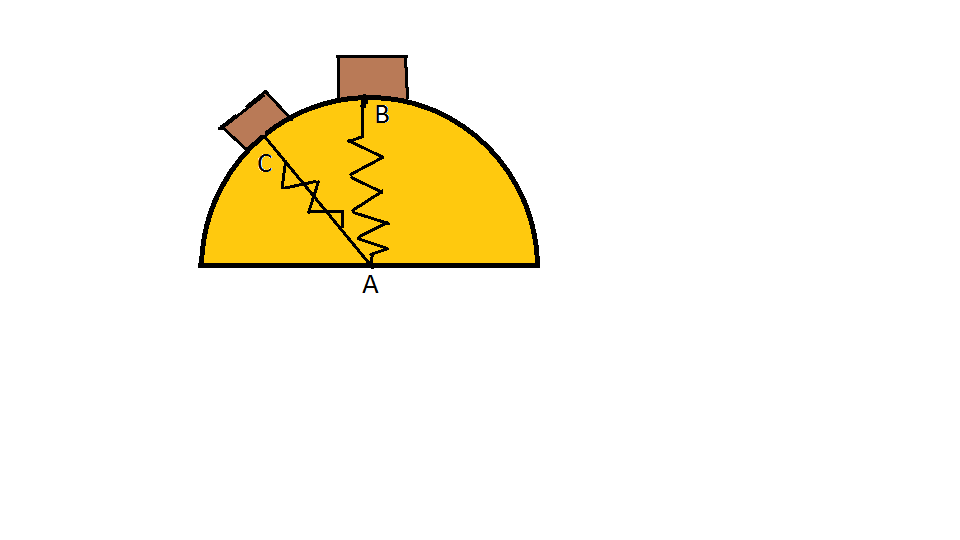# Just do itA 2 kg block is gently pushed from rest at B slides down the smooth circular surface shown in the figure. A spring of strength $k=20$ N/m (attached at the origin of the hemisphere) pulls the block tight against the surface.

What is the unstretched length of the spring (in m) if the spring keeps the block in contact with the surface until the angle with the vertical is 60 degrees?

Assumptions and Details

• The radius of the hemisphere is 1.5 m
• $\angle BAC$ is 60 degrees
×